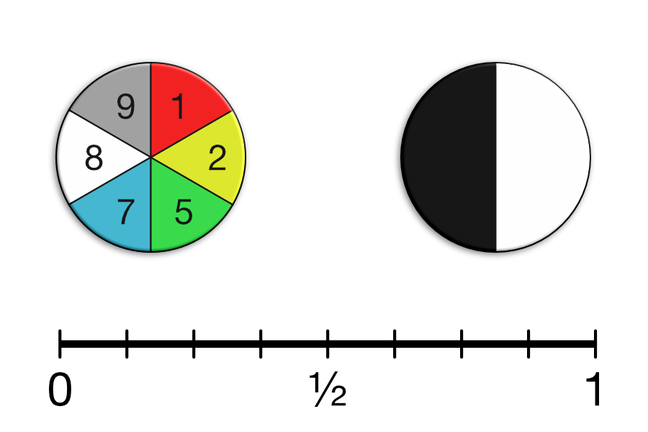## Want to keep learning?

This content is taken from the Cambridge University Press's online course, Teaching Probability. Join the course to learn more.
3.2

## Cambridge University PressSimple outcomes and the probability scale are common examination topics.

# The probability scale

We will start by looking at some of the simpler topics that are typically covered in examinations and assessments:

• The probability scale
• Determining probability using equally likely outcomes
• Understanding fixed probability

In this step we will review the material that students are typically expected to understand for these topics; the next step will present some related questions for you to work through, and will be followed by an opportunity to discuss solutions and approaches.

The probability scale

• The number line above shows a typical representation of the probability scale; students will need to be familiar with the scale presented vertically or horizontally, and labelled using fractions, decimals or percentages.
• Students are sometimes asked to assign verbal descriptions of probability (‘likely’, ‘probable’, etc.) to positions on the probability scale. While this can be a good classroom discussion point, the only descriptions that have unambiguous positions on the scale are ‘impossible’, ‘certain’, and ‘evens’ (or equivalent).

Determining probability using equally likely outcomes

• The simplest type of assessment item involves calculating probabilities given a simple set of discrete outcomes. For example, students may be given a diagram of the numbered spinner shown above, and asked to find the probability of a random spin landing on an odd number.
• More complex questions may involve determining the sample space before finding a probability. For example, students may be asked to complete a table showing the outcomes when both of the spinners shown above are used: each row of the table would show the combinations 1 Black, 1 White, 2 Black, and so on. The question could then ask for the probability of getting (say) any even number combined with Black.

Understanding fixed probability

• This is simply the idea that ‘the apparatus has no memory’. The so-called ‘Gambler’s Fallacy’ is the (wrong) idea that a run of results of one kind means that a different result becomes more likely.
• This is a stubbornly persistent misconception! For example, suppose that the black and white spinner shown above landed on Black five times in a row. Many students (or adults, for that matter) would argue that the next spin is now more likely to land on white.
• This misconception is not entirely irrational; in the long run, results do even out, and we would be very suspicious of a spinner that continued to land on Black every time. The error is to assume that being ‘overdue a White’ somehow has an influence on the result of the next spin. It is the random nature of the process that will cause the results to even out, not some sort of causal feedback based on the previous results.

All clear? Please use the comments to raise any issues that you would like to discuss in more detail.